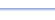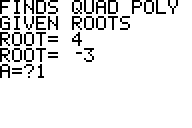• Finds the coefficients of the quadratic polynomial                          , given the roots and the leading coefficient.

Useful for:

• SAT
• ACT
• Algebra 2
• Precalculus

See full list of programs

Example

Q: Find the quadratic polynomial with roots 4 and -3, and with leading coefficient 1.

Solution:

Enter the two roots.

Enter 1 for A (the

The program reports:
These are the
coefficients of the
polynomial.

Interpretation:
The polynomial is